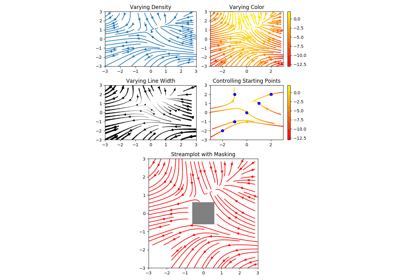/Matplotlib 3.1

# matplotlib.pyplot.streamplot

`matplotlib.pyplot.streamplot(x, y, u, v, density=1, linewidth=None, color=None, cmap=None, norm=None, arrowsize=1, arrowstyle='-|>', minlength=0.1, transform=None, zorder=None, start_points=None, maxlength=4.0, integration_direction='both', *, data=None)` [source]

Draw streamlines of a vector flow.

Parameters: `x, y : 1D arrays` An evenly spaced grid. `u, v : 2D arrays` x and y-velocities. The number of rows and columns must match the length of y and x, respectively. `density : float or (float, float)` Controls the closeness of streamlines. When `density = 1`, the domain is divided into a 30x30 grid. density linearly scales this grid. Each cell in the grid can have, at most, one traversing streamline. For different densities in each direction, use a tuple (density_x, density_y). `linewidth : float or 2D array` The width of the stream lines. With a 2D array the line width can be varied across the grid. The array must have the same shape as u and v. `color : matplotlib color code, or 2D array` The streamline color. If given an array, its values are converted to colors using cmap and norm. The array must have the same shape as u and v. `cmap : Colormap` Colormap used to plot streamlines and arrows. This is only used if color is an array. `norm : Normalize` Normalize object used to scale luminance data to 0, 1. If `None`, stretch (min, max) to (0, 1). This is only used if color is an array. `arrowsize : float` Scaling factor for the arrow size. `arrowstyle : str` Arrow style specification. See `FancyArrowPatch`. `minlength : float` Minimum length of streamline in axes coordinates. `start_points : Nx2 array` Coordinates of starting points for the streamlines in data coordinates (the same coordinates as the x and y arrays). `zorder : int` The zorder of the stream lines and arrows. Artists with lower zorder values are drawn first. `maxlength : float` Maximum length of streamline in axes coordinates. `integration_direction : {'forward', 'backward', 'both'}` Integrate the streamline in forward, backward or both directions. default is `'both'`. `stream_container : StreamplotSet` Container object with attributes `lines`: `LineCollection` of streamlines `arrows`: `PatchCollection` containing `FancyArrowPatch` objects representing the arrows half-way along stream lines. This container will probably change in the future to allow changes to the colormap, alpha, etc. for both lines and arrows, but these changes should be backward compatible.

#### Notes

Note

In addition to the above described arguments, this function can take a data keyword argument. If such a data argument is given, the following arguments are replaced by data[<arg>]:

• All arguments with the following names: 'start_points', 'u', 'v', 'x', 'y'.

Objects passed as data must support item access (`data[<arg>]`) and membership test (`<arg> in data`).

## Examples using `matplotlib.pyplot.streamplot`Streamplot